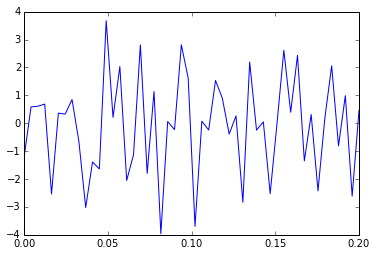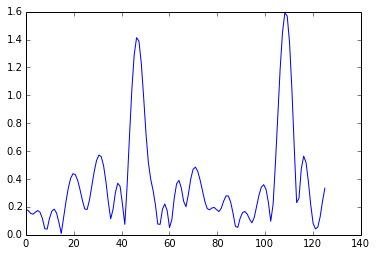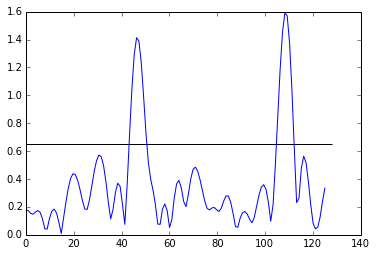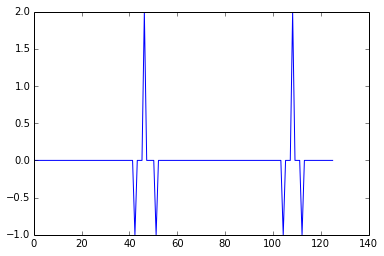# Basic signal processing with IPython Notebook

This IPython notebook revisits a previous example I did in Matlab several years ago. RF signal processing came up as a topic of discussion recenetly, so this example is intended as a refresher/primer and exploration of IPython notebook.

## Prerequisites

In :
%matplotlib inline

In :
from matplotlib.pyplot import *
from numpy import *


Next power of two function. We'll need it later, since the FFT algorithim is only fast for powers of two. That's not important for this example, but in real implementations it needs to execute for every sample window.

In :
def nextpow2(x):
return int(pow(2,ceil(log2(x))))


## Example signal construction

Set the sample frequency and duration.

In :
F_samp = 250
t_max = .2


Values of t are then spaced at interval 1/F_samp.

In :
t = np.matrix(np.linspace(0, t_max, num=F_samp*t_max))


Set the amplitude (A) and frequecny (W) of our example signals.

In :
A = np.matrix("[1.0 1.4]")
W = np.matrix("[45 106]")


Now, construct the signal, including a random noise component. This is the signal that would be observed by the reciever.

In :
sig = A * sin(2*pi*W.T*t) + matrix(random.normal(size=t.shape))

In :
plot(np.squeeze(t.A),np.squeeze(sig.A))

Out:
[<matplotlib.lines.Line2D at 0x10d8ec090>]## Signal detection

Typically, we want to know frequencies of any recieved signals. So, use a fast fourier transform. The FFT algorithim is "fast" when the number of frequency "bins" is a power of two. If we use fewer bins than we have observations, we'll lose information. So, we'll use the next power.

We'll also normalize so that the reported amplitude (y-axis) is in the correct range.

In :
F_max = nextpow2(F_samp)
spec = np.fft.fft(sig, F_max)/(F_samp*t_max)


Create the observed frequency values (x-axis). The Nyquist theorem tells us that the maximum frequency we can observe is half of the sample frequency, so we'll use F_max/2 as the upper limit.

In :
F_obs = F_samp/2*linspace(0, 1, F_max/2)


Also as a consequence of Nyquist, the spectrum returned by the fft is approximately symetric about the y-axis. Typically, we'll only plot the positive half of the graph.

In :
spec_2 = np.squeeze(2*abs( spec[:,0:(F_max/2)] ))


Finally, we'll plot the spectrum. Notice the two peaks corresponding to W with heights similar to A. The height won't match exactly because we've added noise.

In :
plot(F_obs, spec_2);The simplest detection mechinisim is to implement detection as a simple threshold, where the threshold is set to be just above the expected noise. (We can naively guess the threshold by setting A = [0, 0] above and looking looking at this plot. i.e., we're observing background noise when we know that there's no signal.)

In :
thresh = 0.65


We can quickly visualize what part of the spectrum is above the threshold.

In :
plot(F_obs, spec_2);
hlines(thresh,0,F_max/2)

Out:
<matplotlib.collections.LineCollection at 0x10d9734d0>But, we need to mask out the part below the threshold.

In :
spec2_thresh = (spec_2 > thresh) * spec_2


Then we can find zero crossings of the first derivative.

In :
d_spec2_thresh = diff(spec2_thresh)
match = convolve(sign(d_spec2_thresh), [-1,1])
plot(F_obs, match)

Out:
[<matplotlib.lines.Line2D at 0x10da10710>]In :
idx = nonzero(match>0)-2
idx

Out:
array([ 45, 108])

In :
F_obs[idx]

Out:
array([  44.29133858,  106.2992126 ])


Which is close to our original signal W. Note that we corrected the index by two: Once for the element lost in the discrete difference step, and once for the convolution.

In :
W

Out:
matrix([[ 45, 106]])


Now that we have the basics, we're ready to apply these steps to a much larger signal in the next example.